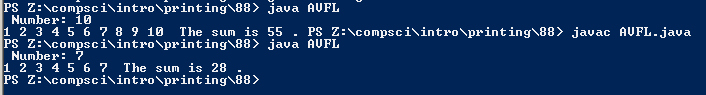# #88

## Code

```  /// Name: Josh Bautista
/// Period: 6
/// Program Name: Adding Values for Loops
/// File Name: AVFL.java
/// Date Finished: 9/2/2015

import java.util.Scanner;

public class AVFL
{
public static void main( String[] args)
{
Scanner keyboard = new Scanner(System.in);
int n1, sum;

System.out.print(" Number: ");
n1 = keyboard.nextInt();
sum = 0;

for ( int n = 1; n <= n1 ; n = n + 1)
{
sum = sum + n;
System.out.print( n + " " );
}
System.out.println(" The sum is " + sum + " . ");
}
}
```

### Picture of the output